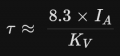# DC Motor Torque

Joined Oct 26, 2012
335
- At a given KV rating, will a motor with higher Watts have more torque?
- At a given watts rating, will a motor with lower KV's have more torque?
- Can we calculate KV from rpm and volts? Eg, 4900rpm/72V=68KV ?

tried to post this in Electromechanical, but couldn't find it

Last edited:

Joined Jul 18, 2013
27,627

•nsaspook

#### crutschow

Joined Mar 14, 2008
32,899
At a given KV rating, will a motor with higher Watts have more torque?
In general, yes.
At a given watts rating, will a motor with lower KV's have more torque?
In general , no.
Can we calculate KV from rpm and volts? Eg, 4900rpm/72V=68KV ?
No.

Joined Oct 26, 2012
335
"At a given watts rating, will a motor with lower KV's have more torque?"
- In general , no.

Hm, i've heard the opposite answer from various sources.

Yes. Until you hit max RPM of course. Lower KV = more torque per amp and lower max RPM.
Hum, maybe you're right:

decreasing the kv manages to increase the torque constant of the motor and if you look at just a torque constant you might think that the lower kV motor makes more torque but as you increase the number of windings you also increase the resistive losses in the windings so the torque constant goes up but the amount of current going through goes down and torque is related directly to current so the total torque actually ends up staying the same

Last edited:

Joined Jul 18, 2013
27,627
Are you using KV in the RC sense?
Krpm/volt?
A BLDC/DC PM motor usually exhibits maximum torque at zero RPM

Joined Oct 26, 2012
335
Are you using KV in the RC sense? Krpm/volt?
yes

A BLDC/DC PM motor usually exhibits maximum torque at zero RPM
Great! So, RPM is part of the torque formula. Can you share the whole formula?

Joined Jul 18, 2013
27,627
RPM is not a Direct factor in torque.
See video in #2

Joined Oct 26, 2012
335
RPM is not a Direct factor in torque.
See video in #2
i have watched the vid, but still trying to understand.
• He mentions $$K_t$$ "torque constant".
• RPM seems related, as it's inversely proportional to torque.
• Also need resistance (i'm assuming that's all kinds of resistance: copper, iron, windings, drive shaft or belt, external friction such as wheel on road etc). For the moment, i'd like to simplify and remove R from the equation.
• V, i assume that means the motor power-supply.
• motor constant seems to be another constant i should learn if i'm trying to select a motor
https://www.celeramotion.com/applim...constant-kt-and-motor-constant-km-of-a-motor/

Joined Oct 26, 2012
335

It took me a while to accept that does indeed equal to KV. Keep in mind that all aspects of an electric motor which impact its KV⋅ (e.g. flux gap size, magnet strength, winding turn number, rotor length etc.) also impact the amount of torque a motor can produce.

Force = Magnetic field ×Current × Conductor length
Increase one of the above terms without negatively impacting another and you have increased the torque output of your system.

There exists a fundamental relationship between an electric motors velocity constant (KV), armature* current (IA) and torque (τ). This relationship is as follows:

τ≈ 8.3×Ia/KVwhere τ is in Nm, IA is in A and KV is in RPM/V.

1. A motors KV and its torque constant (Kτ) are fundamentally the same thing.
2. The torque generated by a motor for a given current is governed by .

This relationship is extremely useful since most 'hobby grade' BLDC/PMSM manufacturers do not publish the usual motor constants.
https://things-in-motion.blogspot.com/2018/12/how-to-estimate-torque-of-bldc-pmsm.html
Now i need to grok that. Also need to understand difference between constant torque, peak torque, acceleration torque....

Last edited: